Question

# A solution is prepared by dissolving 20.2 mLmL of methanol (CH3OH)(CH3OH) in 100.0 mLmL of water...

A solution is prepared by dissolving 20.2 mLmL of methanol (CH3OH)(CH3OH) in 100.0 mLmL of water at 25 ∘C∘C. The final volume of the solution is 118 mLmL. The densities of methanol and water at this temperature are 0.782 g/mLg/mL and 1.00 g/mLg/mL, respectively. For this solution, calculate each of the following.

a. Molarity

b. Molality

C. Percent by mass

d. Mole Fraction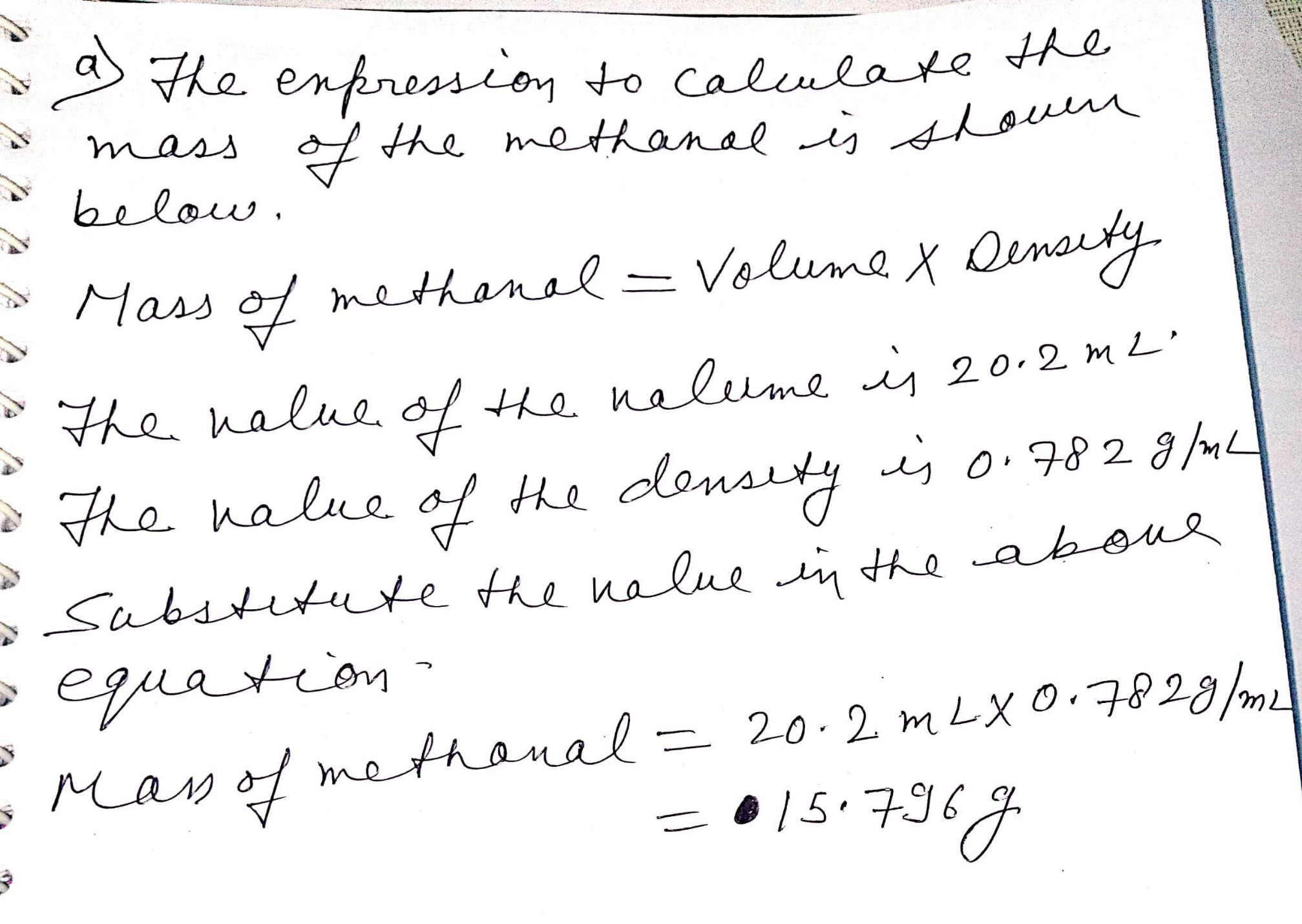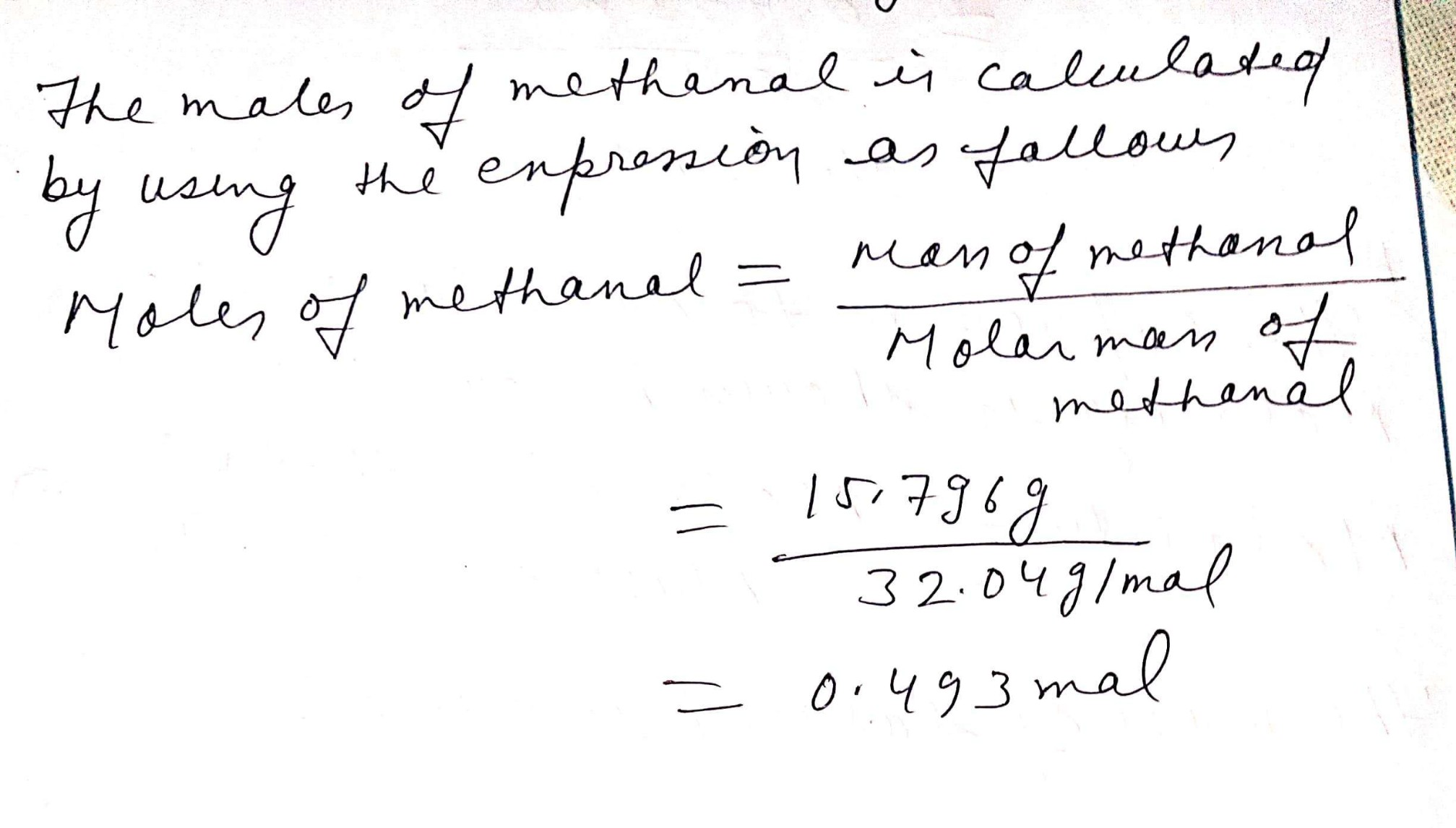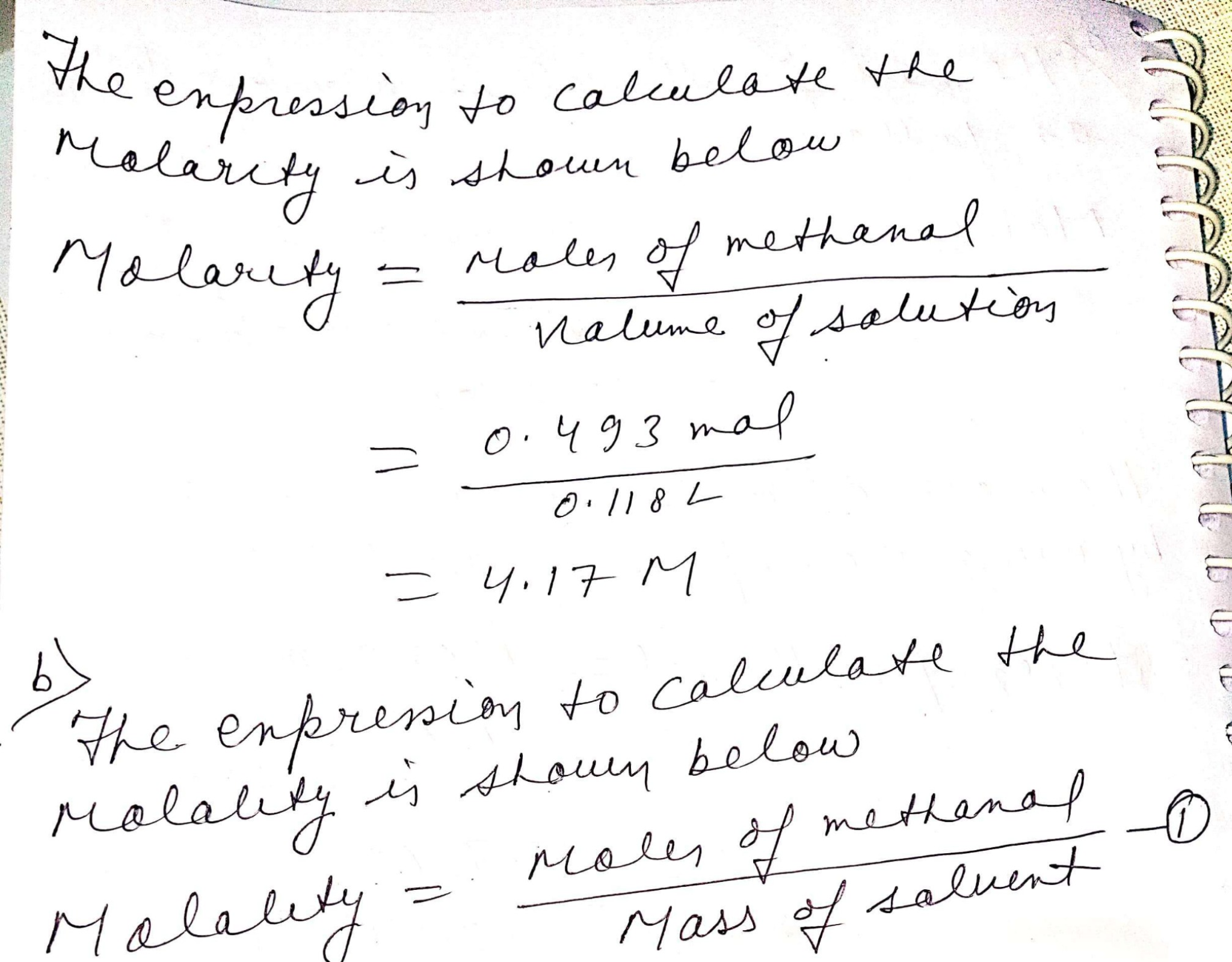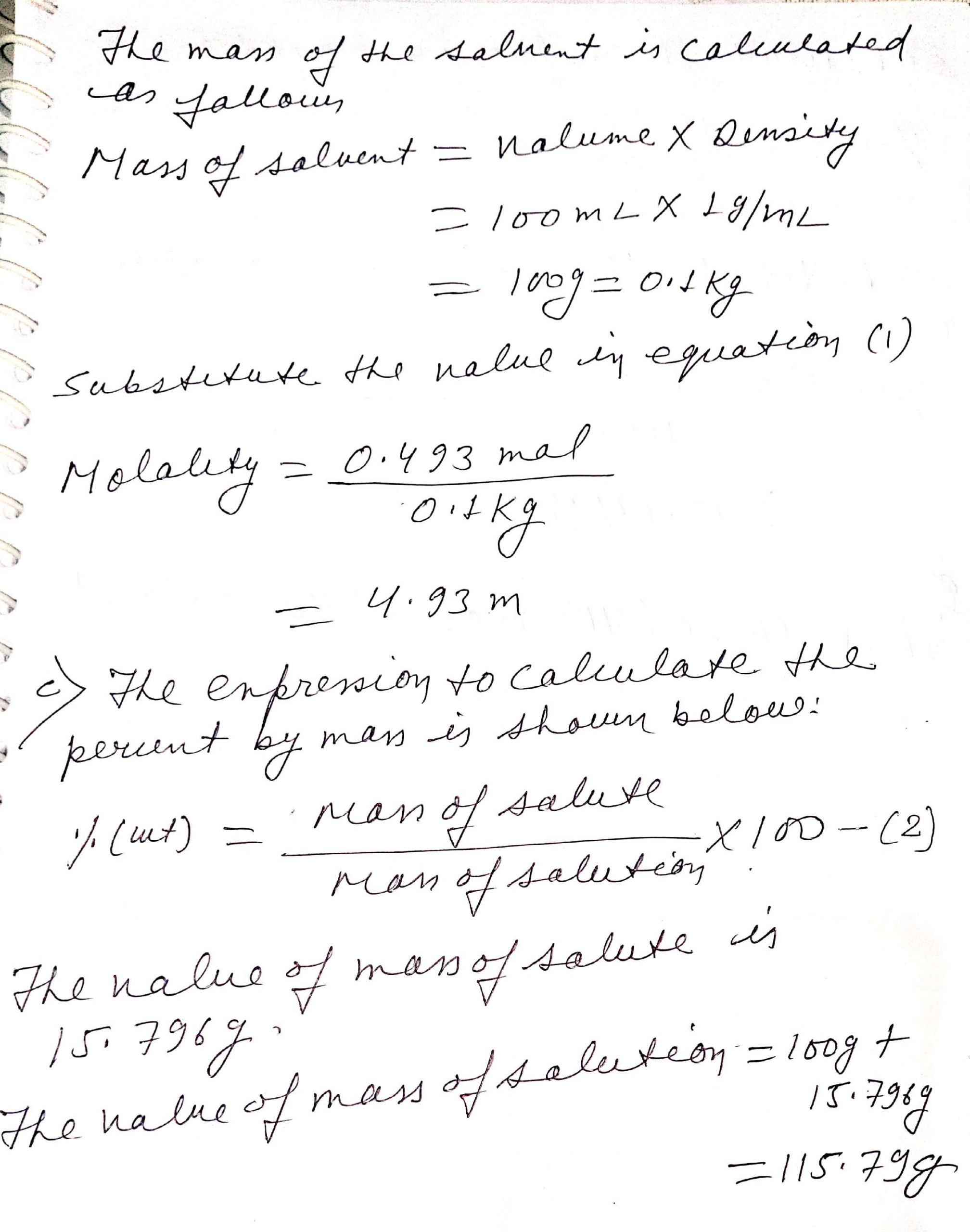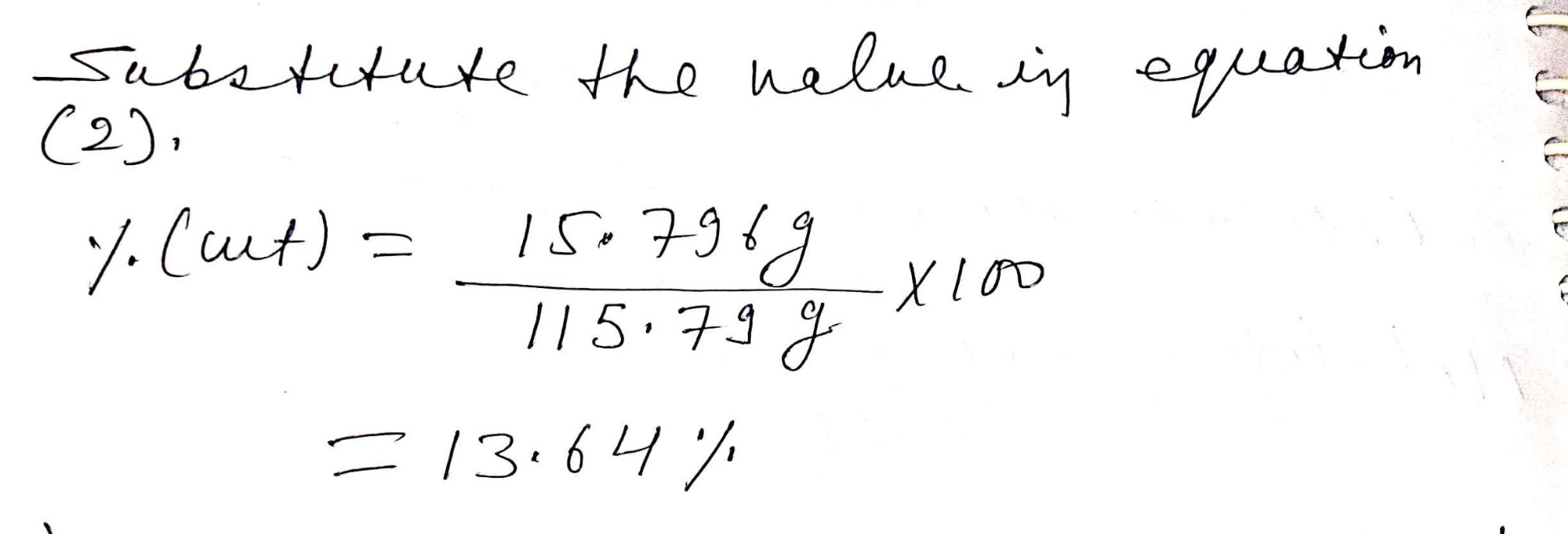#### Earn Coins

Coins can be redeemed for fabulous gifts.

Similar Homework Help Questions
• ### A solution is prepared by dissolving 20.2 mL of methanol (CH3OH) in 100.0 mL of water...

A solution is prepared by dissolving 20.2 mL of methanol (CH3OH) in 100.0 mL of water at 25 ∘C. The final volume of the solution is 118 mL. The densities of methanol and water at this temperature are 0.782 g/mL and 1.00 g/mL, respectively. For this solution, calculate mole percent M=4.18 m=4.93 percent by mass= 13.6% mole fraction= 8.15*10^-2

• ### A solution is prepared by dissolving 21.0mL of methanol in 160.0mL of water at 25 ?C....

A solution is prepared by dissolving 21.0mL of methanol in 160.0mL of water at 25 ?C. The final volume of the solution is 177.9mL . The densities of methanol and water at this temperature are 0.782g/mL and 1.00g/mL , respectively. For this solution, calculate each of the following. Molarity, Molality, percent by mass, mole fraction, and mole percent

• ### 1. A solution is prepared by dissolving 20.2 mL CH,OH in 100.0 mL HO at 25...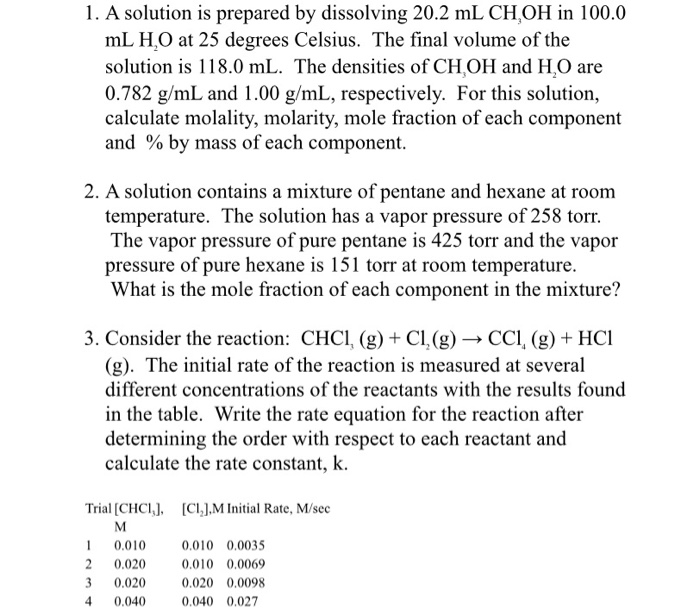1. A solution is prepared by dissolving 20.2 mL CH,OH in 100.0 mL HO at 25 degrees Celsius. The final volume of the solution is 118.0 mL. The densities of CHOH and H,O are 0.782 g/mL and 1.00 g/mL, respectively. For this solution, calculate molality, molarity, mole fraction of each component and % by mass of each component. 2. A solution contains a mixture of pentane and hexane at room temperature. The solution has a vapor pressure of 258 torr....

• ### A solution is prepared by dissolving 50.2 g sucrose (C12H22O11) in 0.383 kg of water. The...

A solution is prepared by dissolving 50.2 g sucrose (C12H22O11) in 0.383 kg of water. The final volume of the solution is 355 mL. (Molarity=0.413, Molality=0.383, and percent by mass is 11.6%) For this solution, calculate mole fraction.

• ### a solution is prepared by dissolving 50.4 g sucrose (c12H22O11) in 0.384 kg of water. The...

a solution is prepared by dissolving 50.4 g sucrose (c12H22O11) in 0.384 kg of water. The final volume of the solution is 355 mL. A. for this solution calculate molarity B. For this solution Calulate molality C. For this solution calculate percent by mass D. For this solution calculate mole fraction

• ### 12. A solution is prepared by dissolving 50.4 g sucrose (CizHzOm) in 0 332 kg of...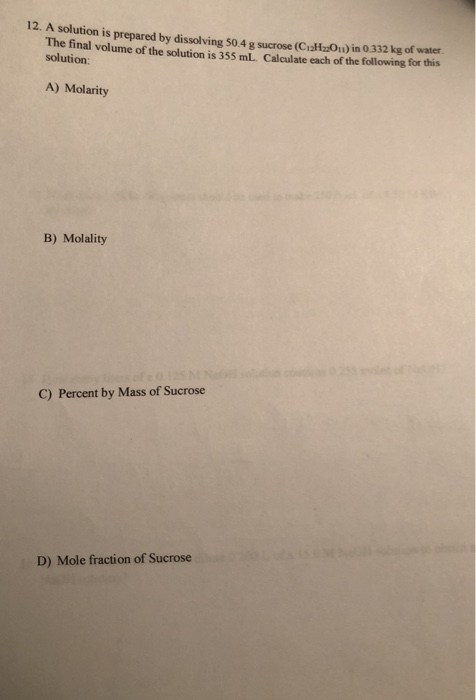12. A solution is prepared by dissolving 50.4 g sucrose (CizHzOm) in 0 332 kg of water The final volume of the solution is 355 mL. Calculate each of the following for this solution: A) Molarity B) Molality C) Percent by Mass of Sucrose D) Mole fraction of Sucrose

• ### A solution was prepared by dissolving 39.0g of KCl in 225 g of water. 1. Calculate...

A solution was prepared by dissolving 39.0g of KCl in 225 g of water. 1. Calculate the mass percent of KCl in the solution. 2. Calculate the mole fraction of KCl in the solution. 3. Calculate the molarity of KCl in the solution if the total volume of the solution is 239 mL. 4. Calculate the molality of KCl in the solution.

• ### A solution is prepared by dissolving 29.2 g of glucose (C6H12O6) in 355 g of water....

A solution is prepared by dissolving 29.2 g of glucose (C6H12O6) in 355 g of water. The final volume of the solution is 376 mL . For this solution, calculate each of the following. A.) molarity B.) moality C. ) percent by mass D.) mole fraction E.) mole percent

• ### Calculate the molality of a solution formed by adding 3.331 g of methanol (CH3OH) to 100.0...

Calculate the molality of a solution formed by adding 3.331 g of methanol (CH3OH) to 100.0 mL of water. The density of water is 0.9982 g/mL.

• ### a solution was prepared by dissolving 27.0 g KOH in 100 g water. A) what is...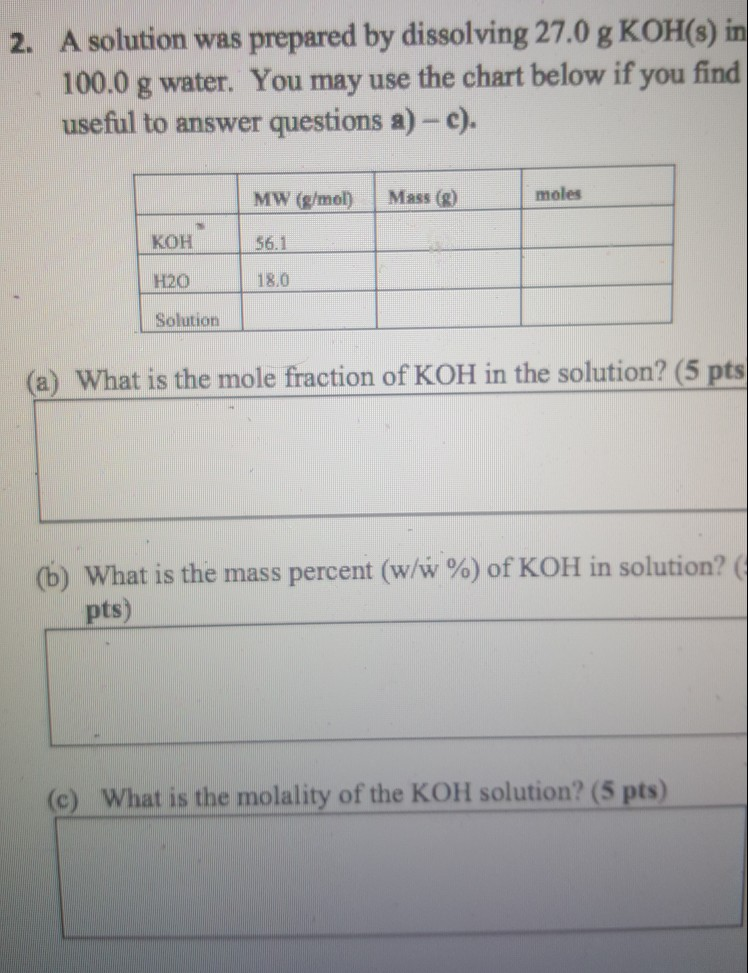a solution was prepared by dissolving 27.0 g KOH in 100 g water. A) what is mole fraction of KOH in solution? B) what is mass percent?C) what is the molality? 2. A solution was prepared by dissolving 27.0 g KOH(s)in 100.0 g water. You may use the chart below if you find usefiul to answer questions a) -e) MW (g/mol Mass ()moles 56.1 H20 18.0 Solution (a) What is the mole fraction of KOH in the solution? (5 pts...